﻿ Coincidence Point Theorem of Two Self-Mappings in Ordered Cone Metric Spaces

### Coincidence Point Theorem of Two Self-Mappings in Ordered Cone Metric Spaces

K. PrudhviOPEN ACCESSPEER-REVIEWED

## Coincidence Point Theorem of Two Self-Mappings in Ordered Cone Metric Spaces

Department of Mathematics, University College of Science, Saifabad, Osmania University, Hyderabad, Telangana State, India.

### Abstract

In the present work, we establish a coincidence point theorem of two mappings in an ordered cone metric spaces, where the cone is not necessarily normal. Our result extends and improves recent results in the literature.

• Prudhvi, K.. "Coincidence Point Theorem of Two Self-Mappings in Ordered Cone Metric Spaces." American Journal of Applied Mathematics and Statistics 2.4 (2014): 249-251.
• Prudhvi, K. (2014). Coincidence Point Theorem of Two Self-Mappings in Ordered Cone Metric Spaces. American Journal of Applied Mathematics and Statistics, 2(4), 249-251.
• Prudhvi, K.. "Coincidence Point Theorem of Two Self-Mappings in Ordered Cone Metric Spaces." American Journal of Applied Mathematics and Statistics 2, no. 4 (2014): 249-251.

 Import into BibTeX Import into EndNote Import into RefMan Import into RefWorks

### 1. Introduction

In 2007, Huang and Zhang  have introduced the concept of cone metric space it is generalization of the metric space. And proved fixed point theorems of contractive type mappings over cone metric spaces. After that, many authors generalized their fixed point theorems to different types of contraction mappings in cone metric spaces (see, [1, 2, 3, 8]). In 2012, Wasfi Shatanawi  proved some coincidence point results in cone metric spaces. In this paper, we prove a coincidence point theorem of two maps in ordered cone metric spaces. Our result extends and improves the results of .

In this paper B is a real Banach space, θ denotes zero element of B.

Definition 1.1 (). Let B be a real Banach Space and P be a subset of B with int(P)≠∅ where P denotes the interior point of P. Then P is called a cone if the following conditions are satisfied:

(a). P is closed, non –empty and P≠{θ};

(b). a,b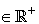, x,y∈P implies ax+by∈P;

(c). x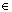P∩ (-P) implies x =θ.

Definition 1.2 (). Let P be a cone in a Banach Space B, define partial ordering ‘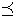’ with respect to P by xy if and only if y-x ∈P. We shall write x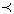y to indicate xy but x≠y while x<<y will stand for y-x∈Int P, where Int P denotes the interior of the set P. This Cone P is called an order cone. It can be easily shown that λ int(P) ⊆int (P) for all λ.

Definition 1.3 (). Let B be a Banach Space and P⊂B be an order cone. The order cone P is called normal if there exists K>0 such that for all x,y∈B,

θxy implies ║x║≤K ║y║.

The least positive number K satisfying the above inequality is called the normal constant of P.

Definition 1.4 (). Let X be a nonempty set of B. Suppose that the map

d: X×X→B satisfies :

(d1).θd(x,y) for all x,y ∈X and

d(x,y) = θ if and only if x = y;

(d2).d(x,y) = d(y,x) for all x,y∈X;

(d3).d(x,y)d(x,z) +d(y,z) for all x,y,z∈X.

Then d is called a cone metric on X and (X, d) is called a cone metric space.

Definition 1.5 (). Let (X,d) be a cone metric space. We say that {xn} is

(i). a Cauchy sequence if for every c in B with

c >>θ, there is N such that for all n, m>N,

d(xn,xm) <<c;

(ii). a convergent sequence if for any

c >>θ, there is an N such that for all n>N,

d(xn, x) <<c, for some fixed x in X.

We denote this xn→x

(as n→∞).

The space (X, d) is called a complete cone metric space if every Cauchy sequence is convergent.

The concept of weakly decreasing maps type A introduce by W. Shatanawi .

Definition 1.6 (). Let (X, ⊆) be partially ordered set and let f, T: X→X be two maps. We say that f is weakly decreasing type A with respect to T if the following conditions hold:

(i). For all x∈X, we have that fx⊆fy for all y∈T-1(fx).

(ii). TX ⊆fX.

Definition 1.7 (). Let (X, d) be a cone metric space and f, g: X→X be two self-maps. The pair {f, g} is said to be compatible if, for an arbitrary sequence {xn}⊂X such that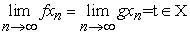, and for arbitrary c∈int (P), there exists n0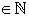such that d(fgxn, gfxn)<< c whenever n > n0. It is said to be weakly compatible if

fx = gx implies fgx = gfx.

Definition 1.8 (). For the mappings f, g: X→X.

If w=fz = gz for some z in X, then z is called a coincidence point of f and g and w is called a point of coincidence of f and g..

### 2. The Main Results

In this section, we prove a coincidence point theorem for two mappings in ordered cone metric spaces without using normal cone. Our result extends and improves the results of .

The following Theorem is extends and improves Theorem 2.2 of .

Theorem 2.1. Let (X, ⊆) be partially ordered set and (X, d) be a complete cone metric space over a solid cone P. Let f, T: X→X be two maps such that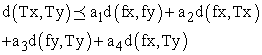(1)

for all x, yX for which fx and fy are comparable. Assume that f and T satisfy the following conditions:

(i). f is weakly decreasing type A with respect to T.

(ii). The pair {f, T} is compatible.

(iii). f and T are continuous.

If ai (i= 1,2,3,4) are non-negative real numbers with a1 + a2 + a3 + 2a4 ∈ [0,1), then f and T have a coincidence point in X, that is there exists a point uX such that fu = Tu.

Proof: Let x0X. Since TX⊆ fX, we can choose x1 ∈X such that Tx0 = fx1. Also since TX⊆ fX, we can choose

x2 ∈X such that Tx1 = f x2. Continuing this process, we can construct a sequence {xn} in X such that Txn= f xn+1. Since xn∈T-1 (f xn+1), n∈N, then by using the assumption that f is weakly decreasing of type A with respect to T,

we have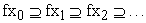By the condition (1) we have,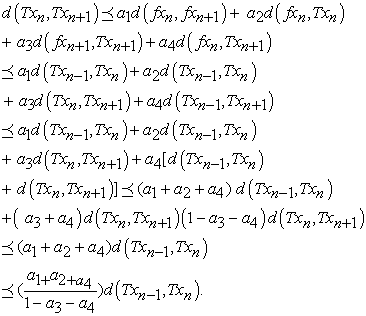Putting,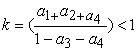.

We obtain,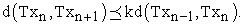(2)

Thus, for n∈N, we have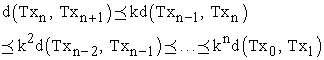Let n, m∈N with m > n. Then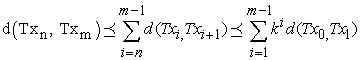Since, k∈ [0,1), we have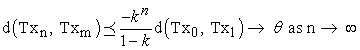(3)

We shall show that {Txn} is a Cauchy sequence in (X, d). For this, let c >> θ be given.

Since, cint(P), then there exists a neighborhood of θ, Nδ(θ) = {yE:║y║< δ},δ>0, such that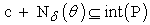Choose a natural number N1 such that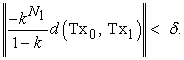Then for all n≥ N1 we have that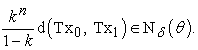Hence, c-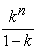d(Tx0, Tx1)c + Nδ(θ) ⊆int(P).

Thus, we have that for all n≥ N1,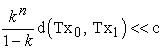(4)

By (3) and (4), it follows that

d(Txn, Txm)<< c whenever n≥ N1.

Hence, {Txn} is a Cauchy sequence in X.

By the completeness of X, there is a

u∈X such that Txn→ u as n→+∞.

Since f and T are continuous, we have

T(Txn) →Tu as n→+ ∞, f (Txn)→fuas n→+ ∞.

By the triangle inequality, we have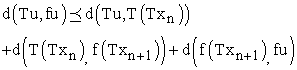(5)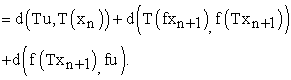(6)

Let θ<<c be given. Then there exists k1 = k1(c) such that d(Tu,T(Txn)) <<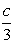for all n≥k1.

Note that, fxn+1 = Txn →u as n→+∞ and Txn+1 →u as n→+∞.

Since {T, f} is compatible, we conclude that there is a

k2 = k2(c) such that d(T(fxn+1), f(Txn+1)) <<for all n ≥ k2.

Finally, there is k3 = k3(c) such that d(T(fxn+1), fu) <<for all n ≥ k3.

Let k0 = max {k1, k2, k3}. By (6) we obtain that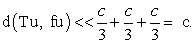Since, c is arbitrary, we conclude that

d(Tu, fu) <<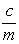for each m∈N. Noting that→ θ as m→∞, we have that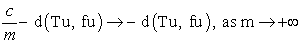Since P is closed, - d (Tu, fu)∈P.

Thus, d(Tu, fu)∈P∩ (-P).

Hence, d (Tu, fu) = θ.

Therefore, f and T have a coincidence point u∈X.

Remark 2.2. If we take a4 = 0 in the above Theorem 2.1, then we get the Theorem 2.2 of .

Remark 2.3. If we take in the above Theorem 2.1

a1 =λ, and a2 = a3 = a4 = 0. Then we can get the following Corollary.

Corollary 2.4. Let (X, ⊆) be partially ordered set and (X, d) be a complete cone metric space over a solid cone P. Let f, T: X→X be two maps such that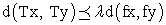(7)

for all x, yX for which fx and fy are comparable. Assume that f and T satisfy the following conditions:

(i). f is weakly decreasing type A with respect to T.

(ii). The pair {f, T} is compatible.

(iii). f and T are continuous.

If λ is a non-negative real numbers with λ∈ [0,1), then f and T have a coincidence point in X.

### References

  M.Abbas and G.Jungck, Common fixed point results for non commuting mappings without continuity in cone metric spaces, J. Math. Anal. Appl. 341 (2008) 416-420.In article CrossRef  I. Altun, G. Durmaz, Some fixed point theorems on ordered cone metric spaces, Rend. Circ. Mat. Palermo 58 (2009) 319-325.In article CrossRef  I. Altun, B.Damjanović, D. Djorić, Fixed point and common fixed point theorems on ordered cone metric spaces, Appl. Math. Lett. 23 (2012) 310-316.In article CrossRef  L.G.Huang, X.Zhang, Cone metric spaces and fixed point theorems of contractive mappings, J. Math. Anal. Appl. 332 (2) (2007) 1468-1476.In article CrossRef  S.Janković, Z.Golubović, S.Radenović, Compatible and weakly compatible mappings in cone metric Spaces, Math. Comput. Modelling 52 (2010) 1728-1738.In article CrossRef  G.Jungck, Compatible mappings and common fixed points, Int. J. Math. Sci. 9 (1986) 771-779.In article CrossRef  G.Jungck, Common fixed point for noncontinuous nonself maps on nonmetric spaces, Far East J. Math. Sci. 4 (1996) 199-215.In article  S.Rezapour and Halbarani, Some notes on the paper “cone metric spaces and fixed point theorem of contractive mappings “, J. Math. Anal. Appl., 345 (2008), 719-724.In article CrossRef  W. Shatanawi, Some coincidence point results in cone metric spaces, Math. Comput. Modeling, Vol. 55, Issue 7-8 (2012) 2023-2028.In article